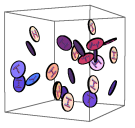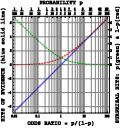# Surprisals, from Excitation to Code

Can a simple strategy for quantifying surprisal provide insight into everyday life?
It has already generated insight into how to: (i) cook food, (ii) make engines do work, (iii) compress data, and (iv) detect kinship between organisms. We suspect that the scope of its applicability will be much broader in the days ahead. Check this out, and let us know what you think...

## Some Surprisal Math

 Quantity Value in {bits, nats, hartleys and J/K} if k is {1/ln2,1,1/ln10,1.38×10-23} respectively Surprisal s[p] = k ln[1/p] ≥ 0 where 0 ≤ p ≤ 1 Evidence for a True-False Assertion e[p] = s[1-p] - s[p] ⇔ odds ratio is 1/2eBits Entropy, Uncertainty, or Information Capacity S = Average[s] = Σipis[pi] ≥ 0 where Σipi = 1 KL-Information or Net Surprisal I = Average[sreference-s] = Σipi(s[poi]-s[pi]) ≥ 0

Surprisal is defined as s = k ln[1/p] where p is a probability (0 ≤ p ≤ 1) and k is a constant that chooses between various unit conventions. For example surprisal is measured in bits if k is 1/ln2~1.44, and in Joules/Kelvin if k is 1.38×10-23. This latter example may seem weird until it's pointed out that reciprocal temperature is energy's uncertainty slope, so that Kelvins measure the "heat energy added per unit increase in entropy". Thus the general inverse relation p=e-s/k becomes this easy-to-remember relation for s in bits: Probability is 1/2#bits. Click [HERE] for some examples of surprisal.

When probabilities multiply, surprisals add. This often makes them easier to work with, and more managable in size, than probabilities. For example, the number of colors possible on a monitor with 256 choices for each of three colors (red, green and blue) is 256 cubed or 16,777,216. This is more simply expressed by pointing out that 8 bits of surprisal for each of three colors results in "24-bits per pixel" altogether. In the special case when there are Ω equally probable alternatives, the fact that probabilities sum to one i.e. Σpi = Sum[pi, {i,1,Ω}] = 1 means that each probability is 1/Ω, and the surprisal associated with each alternative is s = k ln Ω.

Use of surprisals might also help news-media better convey quantitative information to consumers about chances and risk. For example, suppose you plan an action that will reduce the surprisal of you catching smallpox to 16 bits (like that of throwing 16 heads on the first throw of 16 coins). Still not very likely. But if the surprisal of dying from smallpox is only 2 bits (i.e. probability = 1/22 = 1/4), then you might consider getting a vaccination to protect you as long as the surprisal of harm from the vaccination is greater than that of getting done in by smallpox (16+2=18 bits). In practice the surprisal of harm from the vaccine might be closer to 20 bits. The odds of something bad happening either way are tiny, but this simple calculation would let you take informed responsibility for whichever choice you make.

Entropy or uncertainty in information units may be defined as average surprisal S = Σpisi = k Sum[piln[1/pi], {i,1,Ω}]. The name entropy was first used to describe a useful thermodynamic quantity, whose connection to probabilities has been clarified in only the past half century. The underlying idea is that a system, cut off from you and the world, generally does not spontaneously rearrange its state so that the world outside has a more tightly-specified awareness of that state after the fact than before. That is, isolated system entropies in practice don't decrease with time. Put another way, the best guess about the state of an isolated system in the long run is the particular guess which has the most entropy (average surprisal) consistent with the information that you can still count on. Tracking consequences of this assertion mathematically have a long and successful history, clues to which are provided in these notes on the connection between surprisals and thermal physics. Hence then, for example, knowing the energy associated with each state i gives one a ``best guess'' for the final energy. With macroscopic physical systems e.g. with more than 1020 molecules, the resulting predictions can be quite accurate.

In the special case when all Ω alternatives are equally probable, the entropy becomes S = k ln Ω. This logarithmic function of the number of accessible states Ω (#choices=2#bits) is also commonly used to measure the storage capacity of memory devices, that is the amount of information needed to specify its precise state. For example, you might go to the computer store and be told that "To run this program, you need S = 2 gigabytes of RAM (where one byte equals 8 bits)." In that case you need a memory chip that can assume, and hold for later recall, any one of Ω = 28×2,000,000,000 different states. Similarly the uncertainty before choosing one of four answers on a multiple choice test, as well as the information needed to answer correctly, involves 4choices=22bits. How does this change if the correct response might involve multiple choices instead of only one?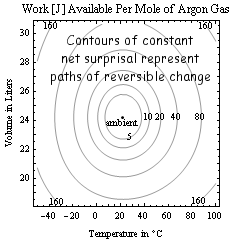Net surprisal (also known as KL-divergence, cross-entropy, and even entropy-deficit although this could mislead) is defined as Inet = Σpi(soi-si) = k Sum[piln[pi/poi], {i,1,Ω}] 0, i.e. as average of the "surprisal-difference" between a reference or "ambient" condition with probabilities denoted poi, and the current state. It also measures the information lost in overlooking differences between a system and the model that you apply to it. Net surprisals are useful in quantifying finite deviations from the reference "equilibrium reservoir" or "model" state in many fields including chemistry, ecology, economics and thermal physics. For example, relative to a room temperature ambient the net surprisal of water near the boiling point is greater than that of water at the freezing point. Thus an invention that converts boiling water to ice water reversibly (i.e. no batteries required) at room temperature is possible, albeit still an engineering challenge. Mutual information (now quite popular in the study of DNA-sequence/chain-letter similarities, quantum computers, and complex non-linear systems) is simply the net surprisal associated with two correlated systems, referenced to the uncorrelated state. Saying that an observer's uncertainty (about an isolated sub-system) does not decrease, as in the discussion of entropies above, may also be seen as an assertion that the mutual information between observer and sub-system is unlikely to increase as long as the isolation persists.

## Some Surprisal Applications

The foregoing equations, although devilishly simple, lend themselves to treatment of a bewildering array of simple and complex, classical and quantum-mechanical, systems. In particular, surprisals in the form of ordered energy (or available work) can be converted into surprisals in the form of subsystem correlations. Two very powerful laws which govern such processes are the first and second laws of thermodynamics. We might refer to the engines that perform such conversions as steady state excitations. Correlations with maximal survivability, on the other hand, often find themselves stored as replicable codes. The perspective of steady state excitations (e.g. organisms which control energy flow) is in some literal sense complementary to that of replicable codes (e.g. genes or texts designed to multiply intact and adapt over time), making this conversion process an interesting balance which fails if run to either extreme (i.e. if rates of energy thermalization are too fast or too slow). For example, if you're starving then energy processing is too slow, whereas if your house is on fire then it's probably too fast.

Life is a symbiosis between such excitations (e.g. organisms) and codes, the latter now quite familiar in both molecular (e.g. genetic) and memetic (e.g. word) form. In this sense both genes and memes are as fundamental to life as the organisms with which they associate. A map that illustrates connections between these things (with interactive links under development) is shown below.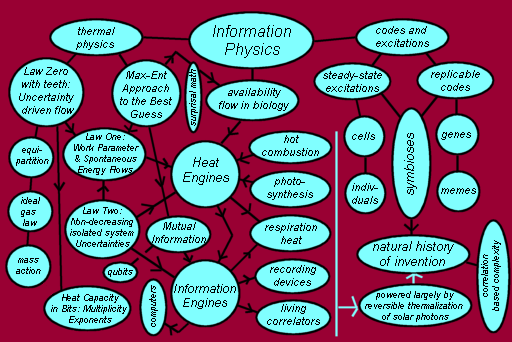The resulting picture (below) of energy flow through steady-state organisms, which thermalize solar energy reversibly with help from molecular codes and memes, may therefore have some interesting quantitative and qualitative uses. This is especially true in light of the great strides that memetic codes have made toward speeding up their own replication in recent decades, e.g. with help from digital hardware and global electronic networks.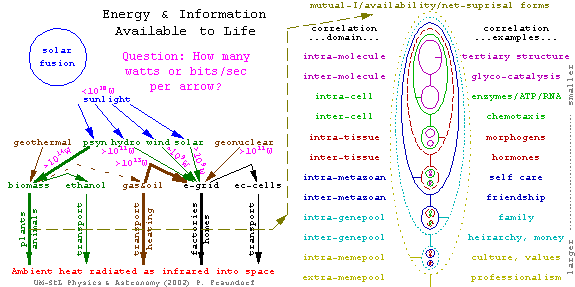Fig. 4 from IFZX: (Left) Life's Energy Flow: The top half represents some of the primary processes involved with energy flow, while the bottom half illustrates significant physical repositories for life's energies, and paths for conversion of energy (and net surprisal) from one form to another. Fig. 5 (Right) Life's Stores of Availability: Horizontal bars represent inward-looking correlations, while vertical bars represent outward-looking correlations. This breakdown seems to work reasonably well to categorize by domain both the types of correlations found, and the types of codes (e.g. genetic or memetic replicators) used to help maintain them.

In the (unfinished) remainder of this note, we plan to explore in more detail how the process of reversible thermalization (i.e. the replacement of ordered energy with correlation information) may be usefully understood as instrumental to many aspects of the natural history of invention...

A primer written for molecular biologists, on logarithms and information theory, may be found here. Surprisal measures are also relevant to:

This page is http://www.umsl.edu/~fraundor/surprise.html. It is hosted by UM-StL Physics and Astronomy, and is Copyright 2003 by P. Fraundorf and collaborators.